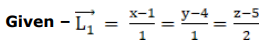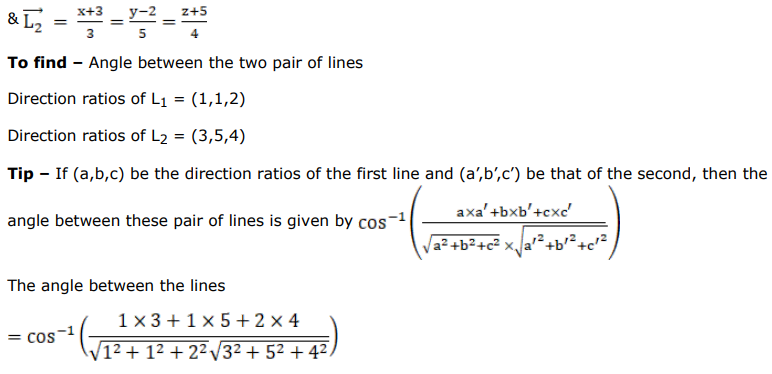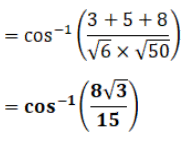# Find the angle between each of the following pairs of lines:

Question:

Find the angle between each of the following pairs of lines:

$\frac{x-1}{1}=\frac{y-4}{1}=\frac{z-5}{2}$ and $\frac{x+3}{3}=\frac{y-2}{5}=\frac{z+5}{4}$

Solution: